Published: 20 April 2018

# Path planning of mobile robot based on hybrid improved artificial fish swarm algorithm

Yi Zhang1
Yuanhong Hua2
1, 2Center of Chongqing Information Accessibility and Service Robot Engineering Technology Research, Chongqing, 400065, China
Corresponding Author:
Yuanhong Hua
Views 182

#### Abstract

The artificial fish swarm algorithm is easy to fall into the local optimum for robot global path planning. A hybrid improved Artificial Fish Swarm Algorithm (HIAFSA) is proposed. Firstly, the sub-optimal path is determined by A* algorithm, and then the adaptive behavior of artificial fish swarm algorithm is improved based on the inertia weight factor, and the attenuation function $\alpha$ is introduced to improve the visual range and moving step length of the artificial fish, balance the global path planning and local path planning, and further improve the convergence speed and quality of the solution. The experimental results show that the hybrid improved artificial fish swarm algorithm has been improved in avoiding local optimum, convergence speed and precision.

## 1. Introduction

With the rapid development of robot technology, mobile service robots are increasingly appearing in people’s daily life. As one of the important manifestations of intelligent mobile robot, autonomous path planning has become the focus of research in the field of robotics, the goal is that the robot moves the robot from the initial position to the target position according to the optimal path or the target (for example, the minimum energy consumption, the better path smoothness, the shortest completion time, etc.), and the movement process To avoid collisions with any obstructions in the environment . At present, there are many algorithms for path planning, such as genetic algorithm, ant colony algorithm, particle swarm algorithm, neural network algorithm . In the natural world, the fish can find the highest food content in the waters according to the behavior of other fish. By analyzing the characteristics of the real fish, the researchers proposed the artificial fish swarm algorithm to construct the artificial fish to simulate the rear tail of the real fish. Group and foraging and other biological behavior activities, the use of bottom-up optimization model to achieve the overall optimization within the work space.

Dr. Li Xiaolei first proposed the algorithm in 2003, and later found that the artificial fish swarm algorithm has strong robustness and fast convergence . The advantages are applicable to the path planning of robots. Peng, Jiansheng maps the path planning to the mathematical model TSP (Traveling Salesman Problem) to improve the detection method of foraging behavior in artificial fish swarm algorithm and improve the convergence efficiency of the algorithm . Wang, Jian et al. Proposed an adaptive behavior-based artificial fish swarm algorithm that ignores the crowding factor and applies it to the robot path planning. Li, Guangqiang et al. Grouped the population into two subgroups of the same size, and applied different adaptation strategies to two groups, one to focus on the global search and the other on the local search . The convergence efficiency and optimization accuracy of the algorithm. Fei, Teng et al. Proposed an improved artificial fish swarm algorithm based on DNA calculation, introducing DNA crossover and DNA mutation operation to improve the slow convergence rate of artificial fish swarm algorithm . Zhang, Chao et al. Proposed an improved artificial fish swarm algorithm to improve the convergence of the algorithm by dynamically adjusting the field of view and step size of artificial fish . The improved artificial fish swarm algorithm proposed above has been effective in improving the convergence and precision of the algorithm, but not taking into account the impact of the size of the artificial fish size on the algorithm and the problem of the global planning and local planning balance of the artificial fish swarm algorithm. The adaptive behavior based on the nonlinear inertia weighting factor $\omega$ is proposed. The attenuation function $\alpha$ is used to dynamically adjust the field of view and the moving step size of the artificial fish, the global optimization and local optimization of the equilibrium algorithm and improve the convergence speed and accuracy of the algorithm.

## 2. Hybrid improvement algorithm description

In AFSA, when the artificial fish population is too large, the algorithm takes a large amount of computation and takes up more storage space. When the fish population is too small, the fish are locally optimized, premature, and easy to fall into the local extreme. In this paper, A* Algorithm  to plan out the second-best path, through the second-best path to determine the available vectors and coordinate points, and then through these different combinations of coordinate points to determine the size of the artificial fish algorithm in the artificial fish. A hybrid improved Artificial Fish Swarm Algorithm (HIAFSA) is proposed. Firstly, the sub-optimal path is determined by A* algorithm, and then the adaptive behavior of artificial fish swarm algorithm is improved based on the inertia weight factor, and the attenuation function $\alpha$ is introduced to improve the visual range and moving step length of the artificial fish, balance the global path planning and local path planning.

### 2.1.1. Artificial fish field of view and step size

In AFSA, if the field of view is too small, the artificial fish will move more randomly. When the field of view is too large, the three adaptive behaviors of the artificial fish will be more prominent but will increase the calculation time. When the artificial fish step, the time to find the optimal path will be reduced, the speed of convergence will be greatly improved, but the step is too large, reduce the artificial fish to find the optimal path to the probability of making it cannot get the optimal solution. In order to improve the visual range and the influence of step value, the attenuation function was introduced to dynamically adjust the visual field and the step length of artificial fish. The attenuation function is as follows:

1
$\alpha =\left(-30\mathrm{*}{\left(\frac{t}{{T}_{max}}\right)}^{s}\right),$

where represents the current number of iterations, represents the maximum number of iterations. When the artificial fish is getting closer and closer to the target, the value of the attenuation function $\alpha$ will get smaller and smaller. In the early stage of the iterative process, the value of is larger, and as the iterative process proceeds, the value of gradually decreases and the attenuation factor is added to the visual field and step size of the artificial fish. Optimal solution and the value of a large problem affecting the convergence rate. Fig. 1 shows the curve of the attenuation function when s takes different values. In HIAFSA, the value of $s$ is 3.

Improved artificial fish vision and steps are as follows:

2
$visual=\frac{{visual}_{min}}{1-\alpha },$
3
$step=\frac{{step}_{min}}{1-\alpha }.$

Which and respectively represent the minimum visual field and the minimum step size.

### 2.1.2. Nonlinear inertia weight factor

In AFSA, artificial fish movement moved to the next position by performing foraging, clustering and tail-catching behaviors, all of which played an important role in the optimization process. Artificial fish foraging behavior, using a random direction, randomness and limitations larger; artificial fish herd behavior is to find the center of vision within the partners, and then to the central location, easy to fall into the local optimal solution; the rear-end behavior of artificial fish is to find the best partner in the field of vision, and then move to it, the conditions for progress is more single. Therefore, in AFSA, the three social behaviors of artificial fish do not balance local planning with global planning. In this paper, three social behaviors of AFSA are dynamically adjusted by inertia weighting factors in Particle Swarm Optimization (PSO) to balance the local path planning with the global path planning. In PSO, the inertia weight factor affects the particle's effective search speed in the current range, which effectively balances the algorithm's ability to develop and explore. The inertia weight factor ω is expressed as follows:

4
$\omega ={\omega }_{max}-\left({\omega }_{max}-{\omega }_{min}\right)\mathrm{*}\mathrm{e}\mathrm{x}\mathrm{p}{\left(-25*\frac{t}{{T}_{max}}\right)}^{3},$

where ${\omega }_{max}$ is the maximum inertia weight, ${\omega }_{min}$ inertia weight minimum. Among them, ${\omega }_{max}=$ 0.9 and ${\omega }_{min}=$ 0.4. The inertia weighting factor $\omega$ decreases non-linearly so that $\omega$ is large and ($1-\omega \right)$ is relatively small in the early iterative process, which helps to improve the global optimization ability of the algorithm. In the whole iteration in the process, $\omega$ will gradually decrease, ($1-\omega \right)$ will increase gradually, improve the latter part of the local optimization ability, balance the global planning and local planning, and solve the conflict between algorithm running time and falling into local optimum.

Improving foraging behavior: Foraging behavior of each artificial fish is relatively independent, introducing a new mathematical expression for foraging behavior by introducing a nonlinear inertia weight factor:

5
${X}_{i/next}={\left(1-\omega \right)X}_{i}+\omega •Rand\left(0,1\right)•step•\frac{{X}_{c}-{X}_{i}}{‖{X}_{c}-{X}_{i}‖}.$

Improved Clustering Behavior: This behavior compares the obtained food concentration ${Y}_{c}$ in the central location with the food concentration ${Y}_{i}$ in the current location. The new mathematical expression of the clustering behavior is as follows:

6
${X}_{i/next}={\left(1-\omega \right)X}_{i}+\omega •Rand\left(0,1\right)•step•\frac{{X}_{c}-{X}_{i}}{‖{X}_{c}-{X}_{i}‖}.$

Improve the rear-end behavior: the behavior of the optimal range of the state of the field ${X}_{max}{Y}_{max}$ concentration of food and the current position of the food concentration ${Y}_{i}$ improve the rear-end behavior of the mathematical expression is as follows:

7
${X}_{i/next}=\left(1-\omega \right){X}_{i}+\omega •Rand\left(0,1\right)•step•\frac{{X}_{max}-{X}_{i}}{‖{X}_{max}-{X}_{i}‖}.$

### 2.2. Hybrid algorithm implementation process

To establish a grid map model, as shown in Fig. 2, a 20×20 grid map , where the black point S(0,0) is the starting point of the mobile robot, the point T(18,15) is the end point;

A* algorithm to plan the next best path, the state recorded in the bulletin board, through the suboptimal path can be marked with some of the path vector ${A}_{1}$, ${A}_{2}$, ${A}_{3}$, ${A}_{4}$, ${A}_{5}$, ${A}_{6}$, ${A}_{7}$ the two endpoints of these vectors as the feasible path of the coordinate point, as shown in Fig. 2 ${a}_{1}$, ${a}_{2}$, ${a}_{3}$ etc. , the latter part of the algorithm is to optimize the adjustment of these coordinate points.

The available coordinate points obtained in the previous step can be arranged and combined to form m feasible paths, for example:$\mathrm{}{a}_{1}{a}_{3}{a}_{4}{a}_{5}{a}_{7\mathrm{}}$, ${a}_{1}{a}_{2}{a}_{3}{a}_{4}{a}_{6}{a}_{7}$, etc. Each path represents an artificial fish. These feasible paths make up the size of the artificial fish population m, setting the congestion factor of the artificial fish population $\delta$; the visual field of the artificial fish “visual”; the maximum number of iterations Artificial fish “Iterate number” Maximum number of attempts to move each iteration “try number”; artificial fish Mobile step

Each artificial fish chooses the best behavior from improved foraging behavior, clustering behavior and rear-end behavior respectively.

The artificial fish, once performed, compares the status of the newly acquired condition with that of the record in the bulletin board, and replaces the status in the bulletin board if the condition is better than that in the bulletin board;

Determine whether the current number of iterations has reached the maximum number of iterations “Iterate number”, if yes, output the results in the bulletin board, otherwise return to the fourth step to continue.

Fig. 1Curve of attenuation function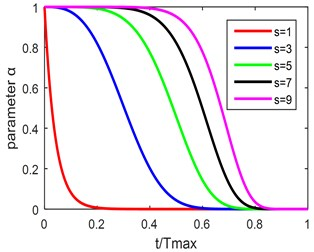Fig. 2Available vectors and coordinate points schematic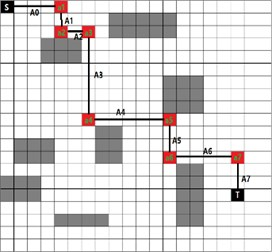## 3. Comparison and analysis of experimental results

In this paper, artificial fish swarm algorithm (AFSA), improved artificial fish school algorithm (IAFSA)  and hybrid improved artificial fish swarm algorithm (HIAFSA) are simulated in two grids with different obstacles. The size of the grid map is 40×40. The point S in the grid map indicates the starting position of the robot, the point T represents the target position of the path planning, and the gray area indicates the obstacle area.

Fig. 3Complex environment grid map 1 and simple environment grid map 2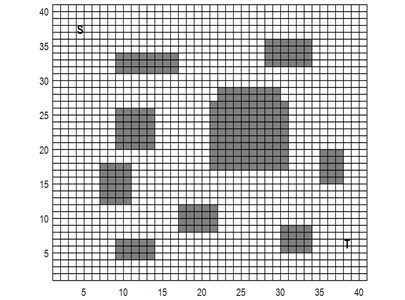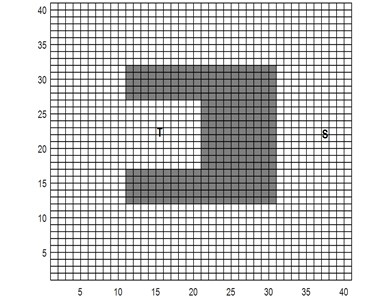As shown in Fig. 3, the grid map simulation environment more complex map, which is characterized by a wide range of obstacles, uneven distribution and irregular size, the robot need to avoid all the obstacles and the optimal path to plan to reach the end. Fig. 4 shows the raster map simulation environment is a simple map, which is characterized by the situation is relatively simple obstacles, the robot needs to go through the corner of 180° reach the end.

Fig. 4 shows the simulation results of the three algorithms in Map 1 and Map 2. The black solid line indicates the path of the HIAFSA plan, the path of the IAFSA and the AFSA plan is indicated by two dotted lines, and the red mark points indicate the available coordinate points generated by the A* algorithm. As can be seen from Fig. 4(b), the optimal path length generated by the three algorithms of map 2 is very close, and the advantage of HIAFSA is not obvious. This is due to the single obstacle in map 2 and the path length of the IAFSA plan is close to the optimal path length, there is not much room for improvement, but for the complex environment of Map 1, the path generated by HIAFSA is significantly shorter. HIAFSA effectively avoids the algorithm getting into local optima, which leads to better path selection in complex environment. The experiment was repeated 50 times, the experimental results in Table 1 statistics. Max and Min in Table 1 represent the maximum and minimum values of the results obtained in 50 experiments, respectively, and Best represents the ideal optimal value. The average time is the average of the 50 repetition tests. The analysis results show that compared with the other two the results of HIAFSA algorithm show that the HIAFSA algorithm has a smaller average path result and is closer to the theoretical optimal value. Meanwhile, the variance of the HIAFSA algorithm is smaller than the other two algorithms. The experimental results show that the HIAFSA algorithm has higher convergence accuracy, more stable, not volatile, the result is more accurate.

Fig. 4Under different maps of three algorithms path planning simulation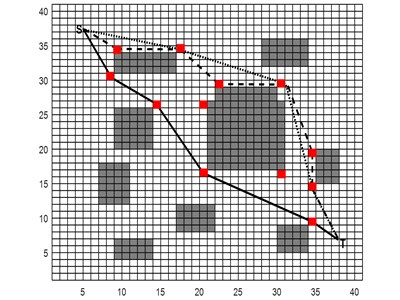a)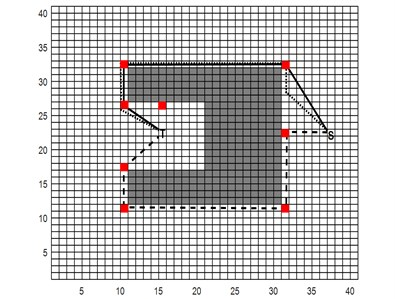b)

Fig. 5Under different maps three algorithms path planning iteration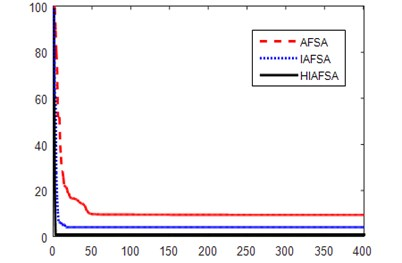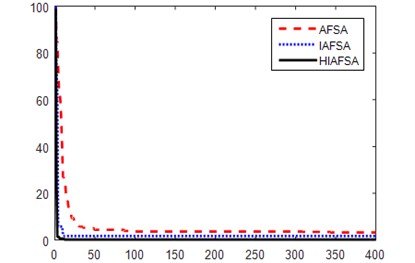Fig. 5 shows the iteration curves of the three algorithms running once on map 1 and map 2, where the ordinate represents the difference between the optimal path generated by the algorithm and the ideal optimal path, the abscissa represents the number of experimental iterations, The results show that the convergence value of HIAFSA algorithm is closer to the ideal optimal value, and it has a faster convergence rate and reaches the global optimum faster.

In summary, the HIAFSA algorithm has the advantages of fast convergence and high convergence precision and has good global optimization and local optimization capabilities. The HIAFSA algorithm has strong applicability and can obtain ideal results for more complicated problems.

Table 1Simulation data

 Number Algorithm name Max Min Best Mean Variance Average time 1 AFSA 71.3145 61.1852 46.4004 66.3863 0.243761 0.762547 IAFSA 62.2054 53.0542 46.4004 57.4571 0.133862 0.695342 HIAFSA 54.2609 47.7286 46.4004 49.0418 0.010581 54.2609 2 AFSA 54.6327 52.1852 48.8062 48.8062 0.0003001 0.658346 IAFSA 52.2768 50.1647 48.8062 48.8062 0.000014 0.553618 HIAFSA 50.2045 49.3023 48.8062 48.8062 0.000000625 0.557364

## 4. Conclusions

In this paper, a hybrid improved artificial fish swarm algorithm is proposed. The A* algorithm and the improved artificial fish swarm algorithm are integrated. the former is used to plan a suboptimal path, and the latter is used to optimize the optimal path so as to obtain the optimal path result, the control parameters of the dynamic adjustment algorithm of attenuation function are introduced, meanwhile the fish population based on nonlinear weighting adaptive behavior is constructed to enhance the global search ability and local search ability of the algorithm in optimization process, and the optimization accuracy of the algorithm is improved; Accuracy and superiority in path planning. the simulation results show that compared with the IAFSA, this algorithm has better quality, faster convergence and more stable convergence, which shows that this algorithm is an effective and improved algorithm.

#### Cited by

A Review of Path Planning for Unmanned Surface Vehicles

26 February 2018
Accepted
11 March 2018
Published
20 April 2018
SUBJECTS
Mathematical models in engineering
Keywords
path planning
A* algorithm
artificial fish swarm algorithm
attenuation function
inertia weight factor
Acknowledgements

This paper belongs to the Project of the “Chongqing Science and Technology Committee, China” No. cstc2015jcyjBX0066 and “Research Project of Chongqing Municipal Education Commission Science and Technology” No. KJ1600442.